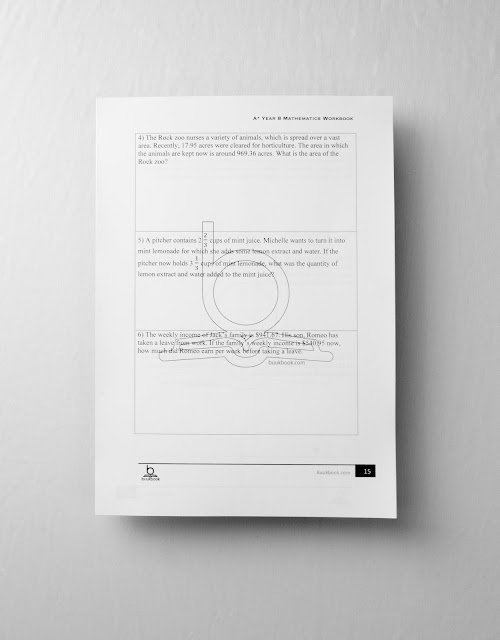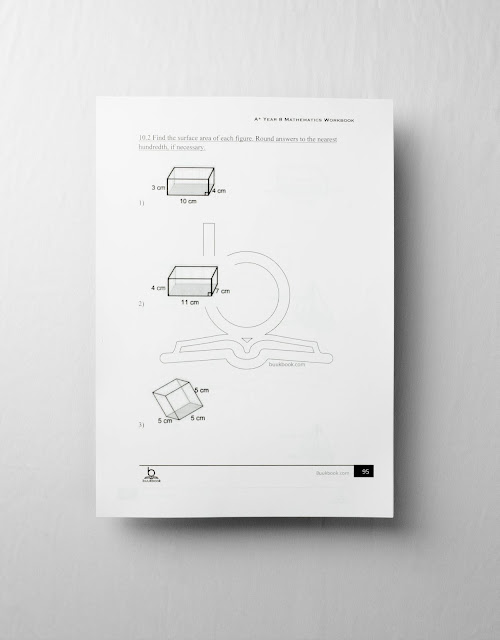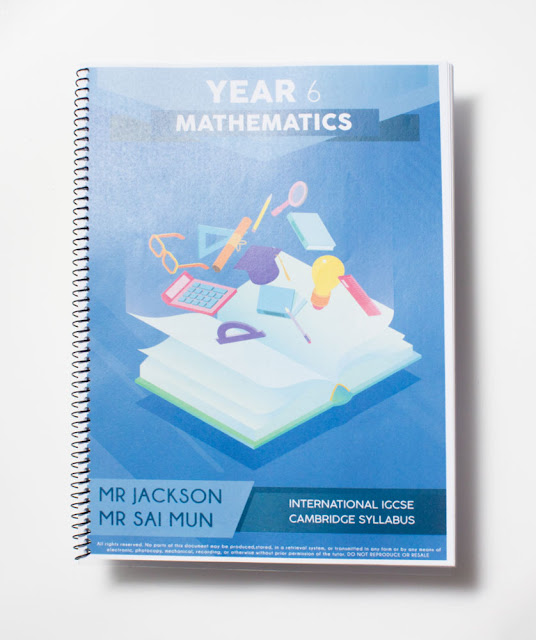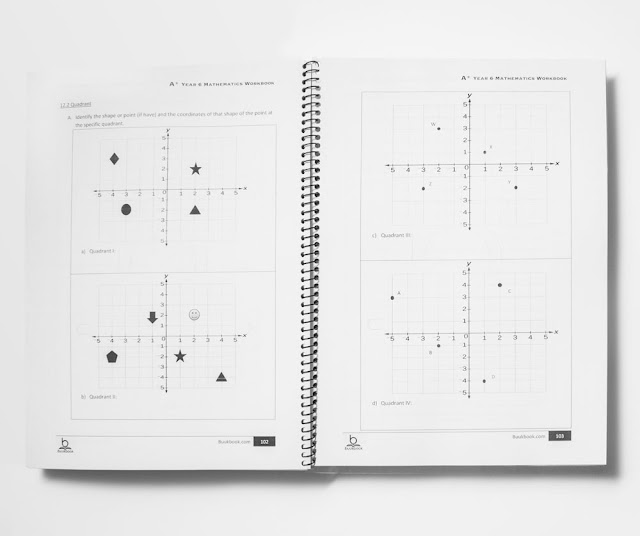# IGCSE A* Workbook

fF

IGCSE A* Workbook Series

Subjects Available:

5.   Year 10 Chemistry
6.   Year 11 Chemistry
7. Year 6 Mathematics

(pls click on the above)

_______________________________________________________________________________

Cambridge IGCSE Year 10
Chemistry Workbook
Version 2.0

Due to our amazing notes and memory booster series, we have received many requests from parents and students to write and design workbooks for international school students.

This workbook will help students to strengthen their year 10 chemistry which is important when they need to do past years in year 11. This workbook will also help teachers to teach and give homework to students while complementing it with IGCSE textbooks.

There are total of 7 chapters in this Year 10 Chemistry A* Workbook:
1. The Particulate Nature of Matter
2. Experimental Techniques
3. Atomic Structure
4. Formulae of Compounds
5. Electrolysis
6. Chemical Energetics
7. Rate of Reaction

well-planned tables and charts with matches IGCSE latest 2017/2018 syllabusStudents can find the answers from IGCSE textbooks when doing self-study revision.
Most suitable for weekly practice and preparation for exam.

Calculations of number of moles definitely need to be drilled.

Learning and Understanding the differences of

Endothermic & Exothermic Reaction at the same time.

Thanks to all the school teachers and parents for their feedback

and has contributed significantly to this very useful workbook.

Marking schemes will be provided for orders of 30 copies and above.

________________________________________________

Cambridge IGCSE Year 11

Chemistry Workbook

There are total of 7 chapters in this Year 11 A* Workbook:

1. Acid, Bases & Salts
2. The Periodic Table
3. Metals
4. Air & Water
5. Sulfur
6. Carbonates
7. Organic Chemistry

Marking schemes will be provided for orders of 30 copies and above.

________________________________________________

Cambridge Year 9
Mathematics Workbook

This math workbook is very useful for teachers and students to drill Mathematics.

This workbook will also help teachers to teach and give homework
to students while complementing it with IGCSE textbooks.

It consists of 12 Chapters:
1. Sequence and Terms
2. Fractions
3. Angles
4. Equations
5. Statistics/ Surveys
6. Measure
7. Powers
8. Graphs
9. Probability
10. Transformation
11. Properties of Numbers and Indices
12. 3-D ShapesAnswer Scheme is provided with the workbook.

________________________________________________

Cambridge Year 7
Mathematics Workbook 1 & 2

This math workbook is very useful for teachers and students to drill Mathematics.
It will also help teachers to teach and give homework to students while complementing it with IGCSE textbooks.

Workbook 1 consists of 12 Chapters

Chapter 1 : Whole Numbers

Chapter 2: Squares, Square Roots and Exponents

Chapter 3 : Factor and Multiples

Chapter 4 : Decimals

Chapter 5 : Fractions

Chapter 6 : Integers

Chapter 7 : Mixed Operations

Chapter 8 : Rational Number

Chapter 9 : Ratio, Rate, and Proportions

Chapter 10 : Percent

Chapter 11 : Unit of Measurements

Chapter 12 : Coordinate Plane

Workbook 2 consists of 9 Chapters

• Chapter 13 : Expression and Properties
• Chapter 14 : Linear Equation
• Chapter 15 : Two-variable Equation
• Chapter 16 : Two Dimensional Figures
• Chapter 17 : Symmetry and Transformation
• Chapter 18 : Construction
• Chapter 19 : Three Dimensional Figures
• Chapter 20 : Geometric Measurement
• Chapter 21 : Data and Graph
Answers are provided with both Mathematics Workbook.

________________________________________________
Cambridge Year 7
Science WorkbookBased on International IGCSE Syllabus, this workbook is suitable for students attending international school Year 7 who are looking to strengthen their science subject with more practice. This book contains structure questions, fill in the blanks, label diagrams, tick correct answers and multiple choice.

Diagrams and formats which can immediately help improve students memory towards the subject and accelerate their learning progress.

CONTENT
Biology
Chapter 1 : Plants
Chapter 2 : Humans as organisms
Chapter 3 : Cells and organism
Chapter 4 : Living things in their environment
Chapter 5 : Variation and classification

Chemistry
Chapter 1 : States of matter
Chapter 2 : Material properties
Chapter 3 : Material changes
Chapter 4 : The earth

Physics
Chapter 1 : Forces
Chapter 2 : Energy
Chapter 3 : The earth and beyond

________________________________________________
Cambridge Year 8

This math workbook is very useful for teachers and students to drill Mathematics.

This workbook will also help teachers to teach and give homework
to students while complementing it with IGCSE textbooks.

It consists of 10 Chapters:
1. Factors, LCM and HCF
2. Algebraic
3. Fraction
4. Ratio
5. Angles
6. Coordinates
7. Transformations
8. Decimals
9. Probability
10. Geometry________________________________________________
Cambridge Year 6

Mathematics WorkbookIt consists of 10 Chapters:
1. Whole Numbers
2. Squares, Square Roots & Exponents
3. Factors and Multiples
4. Decimals
5. Fractions
6. Integers
7. Mixed Operations
8. Rational Number
9. Ratio and Rates
10. Percent
11. Unit of Measurements
12. Coordinate Plane________________________________________________

Other Products

# IGCSE Crash Course Notes# IGCSE Cambridge Year 6 Primary Checkpoint (KS 2)# IGCSE Cambridge Year 9 Secondary Checkpoint (KS 3)________________________________________________

You can purchase from Buukbook.com

sms/whatsapp to 012-718 2825 Ms Soo
017-349 1512 Kenny

012-9413269 Tong

1.all come with mark mark scheme ?

1.yes it comes attach with marking scheme at the back

2.2.Hi .i want the copy.can i buy it??

1.you can order from buukbook.com TQ

3.how can i gei it?

4.HOW CAN I GET YEAR 7 MATHS AND SCIENCE IGCSE

5.hi am interested in buying the year 9 checkpoint pass year paper,
may i know how much you are selling?

6.How much are the books and how do i get them?

7.Hi I interested to buy year 6 checkpoint pass year paper

8.Hi I am interested to purchase year 6 Checkpoint Past Year Paper with model answers.